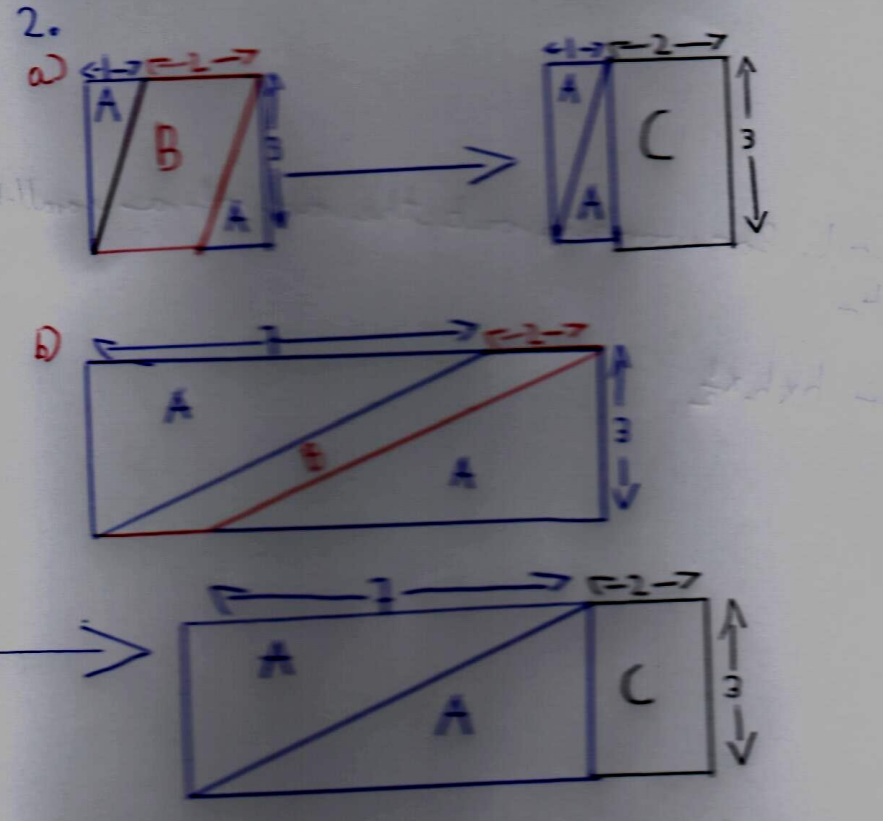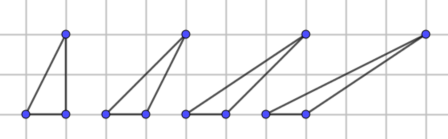#### You may also like### Have You Got It?

Can you explain the strategy for winning this game with any target?### Counting Factors

Is there an efficient way to work out how many factors a large number has?### Is There a Theorem?

Draw a square. A second square of the same size slides around the first always maintaining contact and keeping the same orientation. How far does the dot travel?

# Shear Magic

##### Age 11 to 14Challenge Level

Thank you to everyone who sent in their solutions and ideas for this problem. We received correct answers, or partial answers, from many students around the world including: Aarush, Ronik, Seyon and Yuchen from Wilson's School; Harriet from Romsey School; Helena, Mia, An and Laura from Loughborough High School; Camille from the German European School in Singapore; Emily at The Garden International School in Malaysia; Tessa, Alysia, Hailey, Penny, Matilda, Vihaga and Leia at the Frederick Irwin Anglican School; Tanna and Jazz at Stephen's School Carramar in Australia; Ahan in Singapore; Mahdi from the Mahatma Gandhi International School in India; Sanika at PSBBMS in India; Rose and Pavan (who did not leave their schoools' names) and, Gia and Ken at the British Vietnam International School in Hanoi. Well done to you all.

We began by asking you to share your thoughts about working out the area of Shape C, a parallelogram with base 2 and height 3, and other paralellograms with the same base and height as Shape C.

There are many ways to explain our mathematical thinking to others. This is how Yuchen explained her thinking:

Firstly, I know that the base of picture 1 is 6 and the height is 3 because if we know that base of C is 2 and it takes up 2 squares then we can do the same to the base and height to get 6 and 3.

Now we need to work out the area which is 18 because to work out the area of a rectangle you need to do the length of the base multiplied by the height, which is this case is 6 x 3.

Knowing that the area is 18, we now need to work out the area of A and B. The base for A is 4 and the height is 3, and it is the same for B, which also means the area for both of them is going to be the same, as they are both triangles. Now we do 4 x 3 = 12, however working out the area for a triangle the formula is (base x height) x 1/2. So we do 12 divided by 2, which gives us 6, which means the area for A and B is 6.

Now we do 6 + 6  = 12 and then 18 - 12 = 6 which gives us 6 which means the area for C is also 6.

Well done, Yuchen.

Seyon explored the second part of the question using the following visual approach:Seyon also noticed a pattern in his answers after investigating the areas of several more parallelograms created by shearing rectangles:

I noticed that all the parallelograms that have a base of 2 and a height of 3 have an area of 6. From this I can infer that finding the area of a parallelogram has something to do with both its base and height.

Vihaga and Leia also noticed the importance of knowing a parallelogram's base and height. They generalised their findings as follows:

No matter how stretched out your parallelogram is, the area is always the base multiplied by the height.

Sanika shared the following thoughts:

After I worked out the area of the parallelograms above, I figured that a rectangle was just a unique parallelogram i.e., a parallelogram could be moulded into a rectangle and the area would not change. The rectangle would then have a base that equals the parallelogram's base and a height which equals the height of the parallelogram. The area of the parallelogram would therefore be the area of the rectangle which is nothing but the base of the parallelogram times its height.

Using the GeoGebra applet, I figured that as long as the base and height of the parallelogram were kept constant, the area would not change.

We also encouraged you to investigate what you noticed about a family of sheared triangles

Here is a family of four sheared triangles with a base of 1 and a height of 2:Tanna and Jazz shared their thoughts:

Parallelogram = base x height
Sheared Triangle = base x height x 0.5

This works because a triangle is half the area of a parallelogram.

Matilda wrote:

The rule is that the area of the triangle multiplied by 2 is the area of
the parallelogram.

Are they correct? How do you know?

Sanika explored her ideas further using GeoGebra:

I noticed that the area of a triangle was half the area of a parallelogram which can be formed by diagonally reflecting the triangle. The parallelogram would then have the same height and base as the triangle. Since the area of a parallelogram is base times height, I could say that the area of a triangle would be half its base times height.